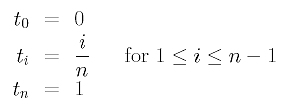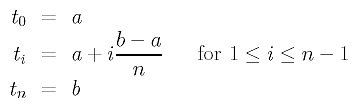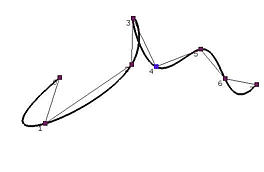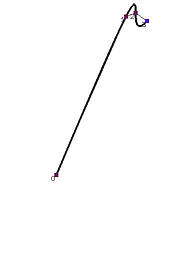# The Uniformly Spaced MethodThe simplest parameter selection method is the uniformly spaced method. Suppose the domain, as usual, is [0,1] and n+1 uniformly spaced parameters are required. The first and the last parameters must be 0 and 1 because we want the curve to pass through the first and the last data points. Therefore, we have t0 = 0 and tn=1.

Since n+1 points divide the interval [0,1] into n subintervals evenly, each of which must be of length 1/n, the division points are 0, 1/n, 2/n, 3/n, ..., (n-1)/n and 1. Thus, we haveFor example, if we need 5 parameters, n = 4, and the uniformly spaced parameters are 0, 1/4, 1/2, 3/4 and 1. If we need 8 parameters, n = 7, and the uniformly spaced parameters are 0, 1/7, 2/7, 3/7, 4/7, 5/7, 6/7 and 1.

What if the domain is [a,b] rather than [0,1]? In this case, [a,b] is divided into n intervals by n+1 division point, a and b included. Since the length of this interval is b - a, the length of each subinterval is (b-a)/n. Hence, the division points (i.e., parameters) areWhile the uniformly spaced method is simple, it is known to generate some unpleasant results. For example, when data points are not uniformly spaced, using uniformly spaced parameters could generate erratic shapes such as big bulges, sharp peaks and loops. In Figure (a) below, there is a loop at data point 3. In Figure (b), the curve wiggles its way through data points 1, 2 and 3. While we cannot say that these problems are unique to the uniformly spaced method, it does occur more frequently than with other methods.(a) (b)